# Texas Go Math Grade 4 Lesson 2.1 Answer Key Model Tenths and Hundredths

Refer to our Texas Go Math Grade 4 Answer Key Pdf to score good marks in the exams. Test yourself by practicing the problems from Texas Go Math Grade 4 Lesson 2.1 Answer Key Model Tenths and Hundredths.

## Texas Go Math Grade 4 Lesson 2.1 Answer Key Model Tenths and Hundredths

Essential Question

How can you model tenths and hundredths?
We know that,
Our system is base ten, a value of 10 in one place is equal to a value of 1 in the place to the left: 10 thousandths are equivalent to 1 hundredth, 10 hundredths are equivalent to 1 tenth, 10 tenths is equivalent to 1 one, and so on.

Investigate

Materials base-ten blocks

A decimal is a number with one or more digits to the right of the decimal point, such as tenths and hundredths. If 1 is divided into ten equal parts, each part is one-tenth. If 1 is divided into one hundred equal parts, each part is one hundredth.

A. What if the small cube is an I unit? Use what you know about the relationships of whole numbers to describe the value of the other blocks.B. What if the flat is I unit? I low can you represent the flat, the lung, and the small cube by using the least number of blocks? the greatest number of blocks? What is the value of each block?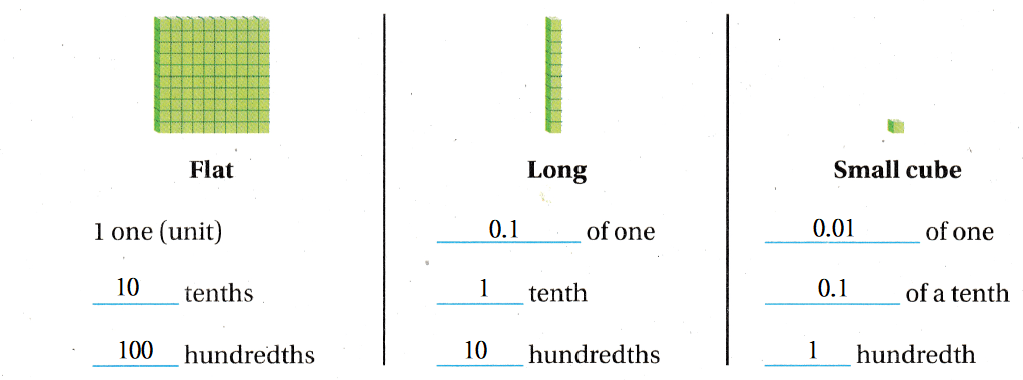C. Model 0.4 with base-ten blocks. Use the flat to represent 1. Tell which blocks you used.
The representation of the model with ten-base blocks is: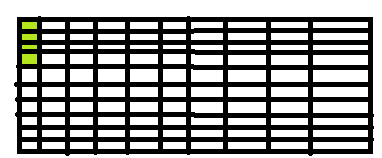Now,
From the above figure,
We can observe that
The blocks you used to represent the ten-base blocks are:
a. Flat
b. Long
c. Cube
Now,
It is given that
The “Flat” is represented as “1”
So,
The “Long” is represented as one-tenth
The “Cube” is represented as one-hundredth
Hence, from the above,
We can conclude that
The value of the representation of the model is: 0.4

Make Connections

You can use your understanding of place-value patterns and a place-value chart to write decimals that are 10 times as much as or $$\frac{1}{10}$$ of any given decimal.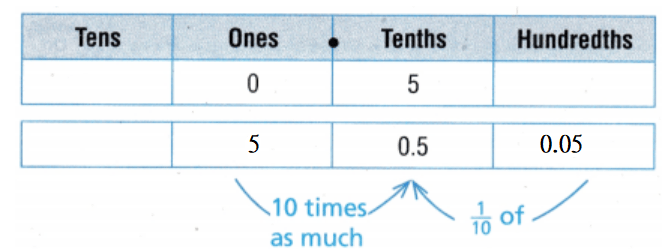5 is 10 times as much as 0.5.
0.05 is $$\frac{1}{10}$$ of 0.5

Use the steps below to complete the table.

STEP 1 Write the given decimal in a place-value chart.
STEP 2 Use the place-value chart to write a decimal that is 10 times as much as the given decimal.
STEP 3 Use the place-value chart to write a decimal that is $$\frac{1}{10}$$ of the given decimal.The given steps are:
STEP 1 Write the given decimal in a place-value chart.
STEP 2 Use the place-value chart to write a decimal that is 10 times as much as the given decimal.
STEP 3 Use the place-value chart to write a decimal that is $$\frac{1}{10}$$ of the given decimal.
Hence, from the above,
We can conclude that
The completed table is: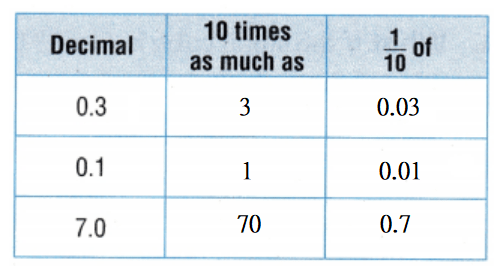Share and Show

Write the decimal shown by the model. The flat represents 1 unit. Then model the decimal in another way. Tell which blocks you used.

Question 1.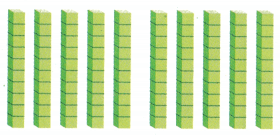The given model is:From the given model,
We can observe that
The model is “Long”
So,
When we considered the “Flat” as 1,
The “Long” is considered as 0.1
So,
The decimal represented by the given model = 0.1 × 10
= 1.0
Hence, from the above,
We can conclude that
The decimal represented by the given model is: 1.0

Question 2.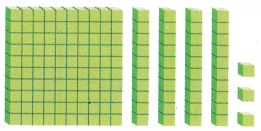The given model is:Now,
From the given model,
We can observe that
The model consists of:
a. Flat
b. Long
c. Cube
Now,
We know that,
When we considered “Flat” as 1,
The “Long” is considered as 0.1 and the “Cube” is considered as 0.1
So,
The decimal represented by the given model = 1 + (0.1 × 4) + (0.01 × 3)
= 1 + 0.4 + 0.03
= 1 + 0.43
= 1.43
Hence, from the above,
We can conclude that
The decimal represented by the given model is: 1.43

Model the decimal in two ways. Use the flat to represent 1. Record by drawing a quick picture.

Question 3.
2.1
The given decimal is: 2.1
Now,
We know that,
We will consider
The “Flat” as 1
The “Long” as 0.1
The “Cube” as 0.01
So,
2.1 = 1 + 1 + 0.1
Hence, from the above,
We can conclude that
The representation of the given decimal number in the form of a model is: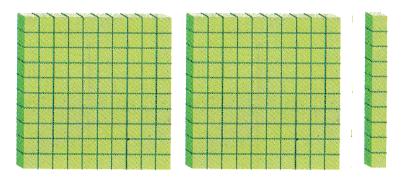Question 4.
0.16
The given decimal is: 0.16
Now,
We know that,
We will consider
The “Flat” as 1
The “Long” as 0.1
The “Cube” as 0.01
So,
0.16 = 0.1 + 0.06
Hence, from the above,
We can conclude that
The representation of the given decimal number in the form of a model is: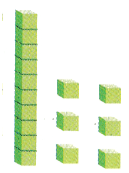Question 5.
3.9
The given decimal is: 3.9
Now,
We know that,
We will consider
The “Flat” as 1
The “Long” as 0.1
The “Cube” as 0.01
So,
3.9 = 1 + 1 + 1 + 0.9
Hence, from the above,
We can conclude that
The representation of the given decimal number in the form of a model is: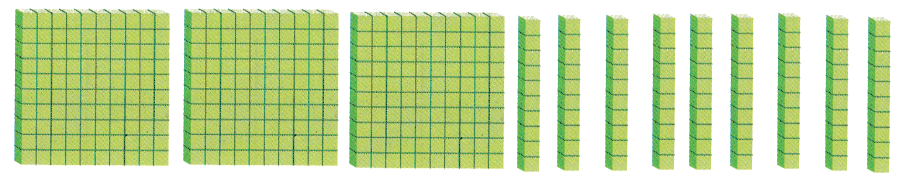Question 6.
H.O.T.
Multi-Step Tyler said he swam 23 tenths miles this week. His coach said Tyler swam 2.3 miles this week.
To find who is correct, model the distances both Tyler and his coach said Tyler swam. Use the flat as 1 unit.

a. What do you need to use?
We need to use the values of the “Flat”, “Long”, and “Cube”

b. What do you know about representing whole numbers and decimals that may help you solve the problem?
We know that,
The value of “Flat” is: 1
The value of “Long” is: 0.1
The value of “Cube” is: 0.01

c. Make a model and draw a quick picture to record the distances that Tyler and his coach said he swam.
It is given that
Tyler said he swam 23 tenths miles this week. His coach said Tyler swam 2.3 miles this week.
Now,
From the given information,
23 tenths = 23 × 0.1
= 2.3
So,
2.3 = 1 + 1 + 0.3
Hence, from the above,
We can conclude that
The model to record the distances that Tyler and his coach said he swam is: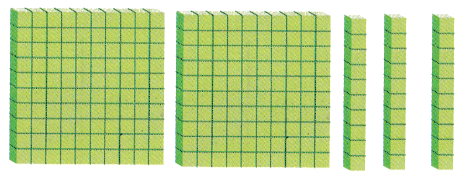d. Complete the sentences.
Are the two models alike or different? ______________________________
Tyler swam __________ tenths, or __________, miles. So, ______________________________ are correct.
The two models are alike
Tyler swam 23 tenths or 2.3 miles
So,
Both Tyler and his coach are correct

Question 7.
Multi-Step:
Using Diagrams Mike and Shantel both drew a quick picture to represent the decimal 1.2. Whose quick picture is correct? Explain the error that either Mike or Shantel made when drawing their quick picture.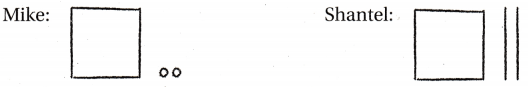It is given that
Mike and Shantel both drew a quick picture to represent the decimal 1.2
Now,
We know that,
We will consider “Flat” as 1
We will consider “Long” as: 0.1
We will consider “Cube” as: 0.01
Now,
The model of Mike is: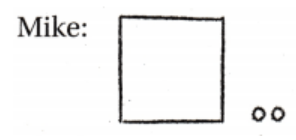Now,
From the above model,
The representation of the decimal number = 1 + (2 × 0.01)
= 1 + 0.02
= 1.02
Now,
The model of Shantel is:Now,
From the above model,
The representation of the decimal number = 1 + (2 × 0.1)
= 1 + 0.2
= 1.2
Hence, from the above,
We can conclude that
Shantel’s quick picture is correct
Mike considered the value of “Cube” as 0.1 which is the error

Question 8.
Representations In the models below, the flat represents 1 unit. The width of an apple seed is 0.31 centimeters. Which model shows 0.31?
(A)(B)(C)(D)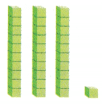It is given that
The width of an apple seed is 0.31 centimeters
Now,
We know that,
The value of “Flat” is: 1
The value of “Long” is: 0.1
The value of “Cube” is: 0.01
So,
0.31 = 0.3 + 0.01
= (0.1 × 3) + 0.01
= 3 Long + 1 Cube
Hence, from the above,
We can conclude that
The model that shows 0.31 is:Question 9.
Multi-Step In the model below, the flat represents 1 unit. Which is another way to model this decimal?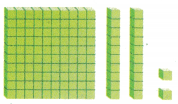(A)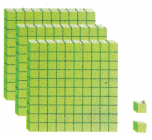(B)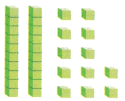(C)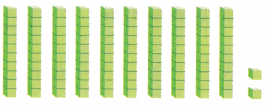(D)The given model is:Now,
We know that,
The value of “Flat” is: 1
The value of “Long” is: 0.1
The value of “Cube” is: 0.01
Now,
From the given model,
We can observe that
The representation of the decimal number = 1 + (2 × 0.1) + (2 × 0.01)
= 1 + 0.2 + 0.02
= 1.2 + 0.02
= 1.22
= (12 ×0.1) + (2 × 0.01)
= 12 Long + 2 Cubes
Hence, from the above,
We can conclude that
The another way to model the given model is:TEXAS Test Prep

Question 10.
Suppose the flat represents 1 unit. What decimal is represented by the model?(A) 62
(B) 0.62
(C) 6.2
(D) 0.26
The given model is:Now,
We know that,
The value of “Flat” is: 1
The value of “Long” is: 0.1
The value of “Cube” is: 0.01
Now,
From the given model,
We can observe that
The representation of the decimal number = (6 × 0.1) + (2 × 0.01)
= 0.6 + 0.02
= 0.62
Hence, from the above,
We can conclude that
The decimal number that is represented by the given model is:### Texas Go Math Grade 4 Lesson 2.1 Homework and Practice Answer Key

Model Tenths and Hundredths

Question 1.
Lucia and Stephen each drew a quick picture to model 2.04. Whose model is correct? Explain the error.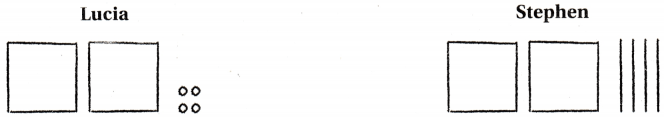It is given that
Lucia and Stephen each drew a quick picture to model 2.04
Now,
We know that,
We will consider “Face” as: 1
We will consider “Long” as: 0.1
We will consider “Cube” as: 0.01
Now,
The model of Lucia is:Now,
From the above model,
We can observe that
The decimal number represented by the above model = 1 + 1 + (4 × 0.01)
= 2 + 0.04
= 2.04
Now,
The model of Stephen is: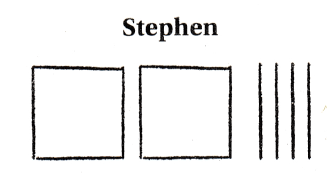Now,
From the above model,
We can observe that
The decimal number represented by the above model = 1 + 1 + (4 × 0.1)
= 2 + 0.4
= 2.4
Hence, from the above,
We can conclude that
Lucia’s model is correct
The error is that Stephen considered “Long” instead of “Cube”

Problem Solving

Question 2.
Russell planted a flower bed that is 1.6 square meters. Jackie planted a flower bed that is 16 tenths square meters. Draw a quick picture to model the two areas.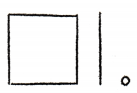Russell                                  Jackie
It is given that
Russell planted a flower bed that is 1.6 square meters. Jackie planted a flower bed that is 16 tenths square meters
Now,
We know that,
We will consider “Face” as: 1
We will consider “Long” as: 0.1
We will consider “Cube” as: 0.01
Hence, from the above,
We can conclude that
The representation of the area models of Russel and Jackie is:
Russel:Jackie: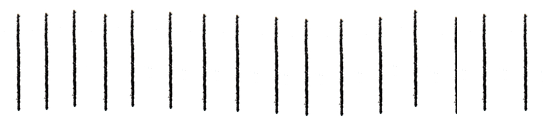Question 3.
Compare the areas of the two flower beds. Explain your reasoning.
From Question 2,
It is given that
Russell planted a flower bed that is 1.6 square meters. Jackie planted a flower bed that is 16 tenths square meters
So,
16 tenths = 16 × 0.1
= 1.6
So,
1.6 = 1.6
Hence, from the above,
We can conclude that
The area models of Russel and Jackie are the same

Lesson Check

Question 4.
In the model below, the flat shows 1 unit. What decimal does the model show?(A) 3.5
(B) 0.53
(C) 0.35
(D) 3.05
The given model is:Now,
We know that,
We will consider “Face” as: 1
We will consider “Long” as: 0.1
We will consider “Cube” as: 0.01
Now,
From the above figure,
We can observe that
The decimal number represented by the given model = 1 + 1 + 1 + (5 × 0.01)
= 3 + 0.05
= 3.05
Hence, from the above,
We can conclude that
The decimal number represented by the given model is:Question 5.
In the models below, the flat shows 1 unit. Which model shows 0.24?
(A)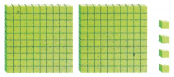(B)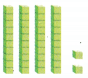(C)(D)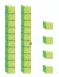The given decimal number is: 0.24
Now,
We know that,
We will consider “Face” as: 1
We will consider “Long” as: 0.1
We will consider “Cube” as: 0.01
So,
0.24 = 0.2 + 0.04
= ( 2 × 0.1) + (4 × 0.01)
= 2 Long + 4 Cube
Hence, from the above,
We can conclude that
The representation of the model for the given decimal number is:Question 6.
Multi-Step In the model below, the flat represents 1 unit. Which is another way to model this decimal?(A)(B)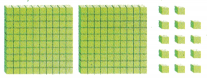(C)(D)The given model is:Now,
We know that,
We will consider “Face” as: 1
We will consider “Long” as: 0.1
We will consider “Cube” as: 0.01
Now,
From the above model,
We can observe that
The representation of the decimal number for the given model = 1 + (3 × 0.1) + (4 × 0.01)
= 1 + 0.3 + 0.04
= 1 + 0.34
= 1.34
= (10 × 0.1) + (3 × 0.1) + (4 × 0.01)
= (13 × 0.1) + (4 × 0.01)
= 13 Long + 4 Cube
Hence, from the above,
We can conclude that
The another way to model the given model is: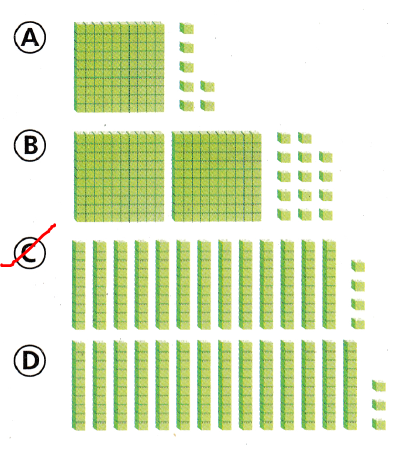Question 7.
In the model below, the flat shows 1 unit. What are the fraction and decimals shown by the model?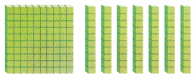(A) 1.7, 1$$\frac{7}{10}$$
(B) 1.7, 1$$\frac{1}{7}$$
(C) 0.17, $$\frac{17}{100}$$
(D) 1.07, 1$$\frac{7}{100}$$
The given model is:Now,
We know that,
We will consider “Face” as: 1
We will consider “Long” as: 0.1
We will consider “Cube” as: 0.01
Now,
From the above model,
We can observe that
The representation of the decimal number for the given model = 1 + (7 × 0.1)
= 1 + 0.7
= 1.7
= $$\frac{17}{10}$$
= 1$$\frac{7}{10}$$
Hence, from the above,
We can conclude that
The fraction and the decimal number shown by the given model is: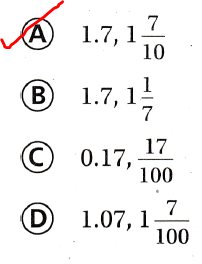Scroll to Top